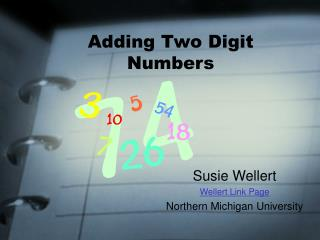DownloadDownload PresentationDownload Presentation- - - - - - - - - - - - - - - - - - - - - - - - - - - E N D - - - - - - - - - - - - - - - - - - - - - - - - - - -
##### Presentation Transcript

1. Adding Two Digit Numbers Susie Wellert Wellert Link Page Northern Michigan University

2. Adding Multiples of Ten This is how we show 10+10=20: Each bundle of sticks equals 10 so two bundles of 10 equals 20.

3. Continued Here is another way that we can show 10+10=20: Click here for some practice.

4. Expanded Form What number is this? It is the number 34. We are going to write 34 in a new way now. How many are in the tens? There are 3 tens, that equals 30. Plus how many ones? There are 4 ones. When you add them together they make 34.

5. Expanded Form Continued So now we can write 34 in a new way… 34 = 3 tens + 4 34 = 30 + 4 34 = 30 + 4 is called expanded form. Click here to do a worksheet for practice.

6. Simplest Form Do you know what the simplest name is for the answer to this: 40 + 7 = ___ The simplest name is 47.

7. Putting It All Together Now we have all the pieces that we need to add two digit numbers. Just follow the example below: All you have to do is add the bundles of sticks together then add the tally marks together to find how many are in all.

8. Now you know how to add two digit numbers!! Click here to take the quiz. To check your answers click on each of these, worksheet1, worksheet2 and the quiz.